# Finite summation formulas involving binomial coefficients, harmonic numbers and generalized harmonic numbers

## Abstract

A variety of identities involving harmonic numbers and generalized harmonic numbers have been investigated since the distant past and involved in a wide range of diverse fields such as analysis of algorithms in computer science, various branches of number theory, elementary particle physics and theoretical physics. Here we show how one can obtain further interesting identities about certain finite series involving binomial coefficients, harmonic numbers and generalized harmonic numbers by applying the usual differential operator to a known identity.

MSC:11M06, 33B15, 33E20, 11M35, 11M41, 40C15.

## 1 Introduction and preliminaries

The generalized harmonic numbers ${H}_{n}^{\left(s\right)}$ of order s are defined by (cf. ; see also [2, 3], [, p.156] and [, Section 3.5])

${H}_{n}^{\left(s\right)}:=\sum _{j=1}^{n}\frac{1}{{j}^{s}}\phantom{\rule{1em}{0ex}}\left(n\in \mathbb{N};s\in \mathbb{C}\right),$
(1.1)

and

${H}_{n}:={H}_{n}^{\left(1\right)}=\sum _{j=1}^{n}\frac{1}{j}\phantom{\rule{1em}{0ex}}\left(n\in \mathbb{N}\right)$
(1.2)

are the harmonic numbers. Here and denote the set of positive integers and the set of complex numbers, respectively, and we assume that

${H}_{0}:=0,\phantom{\rule{2em}{0ex}}{H}_{0}^{\left(s\right)}:=0\phantom{\rule{1em}{0ex}}\left(s\in \mathbb{C}\setminus \left\{0\right\}\right)\phantom{\rule{1em}{0ex}}\text{and}\phantom{\rule{1em}{0ex}}{H}_{0}^{\left(0\right)}:=1.$

The generalized harmonic functions ${H}_{n}^{\left(s\right)}\left(z\right)$ are defined by (see [2, 6]; see also [7, 8])

${H}_{n}^{\left(s\right)}\left(z\right):=\sum _{j=1}^{n}\frac{1}{{\left(j+z\right)}^{s}}\phantom{\rule{1em}{0ex}}\left(n\in \mathbb{N};s\in \mathbb{C}\setminus {\mathbb{Z}}^{-};{\mathbb{Z}}^{-}:=\left\{-1,-2,-3,\dots \right\}\right)$
(1.3)

so that, obviously,

${H}_{n}^{\left(s\right)}\left(0\right)={H}_{n}^{\left(s\right)}.$

Equation (1.1) can be written in the following form:

${H}_{n}^{\left(s\right)}=\zeta \left(s\right)-\zeta \left(s,n+1\right)\phantom{\rule{1em}{0ex}}\left(\mathrm{\Re }\left(s\right)>1;n\in \mathbb{N}\right)$
(1.4)

by recalling the well-known (easily-derivable) relationship between the Riemann zeta function $\zeta \left(s\right)$ and the Hurwitz (or generalized) zeta function $\zeta \left(s,a\right)$ (see [, Eq. 2.3(9)])

$\zeta \left(s\right)=\zeta \left(s,n+1\right)+\sum _{k=1}^{n}{k}^{-s}\phantom{\rule{1em}{0ex}}\left(n\in {\mathbb{N}}_{0}:=\mathbb{N}\cup \left\{0\right\}\right).$
(1.5)

The polygamma functions ${\psi }^{\left(n\right)}\left(s\right)$ ($n\in \mathbb{N}$) are defined by

${\psi }^{\left(n\right)}\left(s\right):=\frac{{d}^{n+1}}{d{z}^{n+1}}log\mathrm{\Gamma }\left(s\right)=\frac{{d}^{n}}{d{s}^{n}}\psi \left(s\right)\phantom{\rule{1em}{0ex}}\left(n\in {\mathbb{N}}_{0};s\in \mathbb{C}\setminus {\mathbb{Z}}_{0}^{-}:={\mathbb{Z}}^{-}\cup \left\{0\right\}\right),$
(1.6)

where $\mathrm{\Gamma }\left(s\right)$ is the familiar gamma function, and the psi-function ψ is defined by

$\psi \left(s\right):=\frac{d}{ds}log\mathrm{\Gamma }\left(s\right)\phantom{\rule{1em}{0ex}}\text{and}\phantom{\rule{1em}{0ex}}{\psi }^{\left(0\right)}\left(s\right)=\psi \left(s\right)\phantom{\rule{1em}{0ex}}\left(s\in \mathbb{C}\setminus {\mathbb{Z}}_{0}^{-}\right).$

A well-known (and potentially useful) relationship between the polygamma functions ${\psi }^{\left(n\right)}\left(s\right)$ and the generalized zeta function $\zeta \left(s,a\right)$ is given by

${\psi }^{\left(n\right)}\left(s\right)={\left(-1\right)}^{n+1}n!\sum _{k=0}^{\mathrm{\infty }}\frac{1}{{\left(k+s\right)}^{n+1}}={\left(-1\right)}^{n+1}n!\zeta \left(n+1,s\right)\phantom{\rule{1em}{0ex}}\left(n\in \mathbb{N};s\in \mathbb{C}\setminus {\mathbb{Z}}_{0}^{-}\right).$
(1.7)

It is also easy to have the following expression (cf. [, Eq. 1.2(54)]):

${\psi }^{\left(m\right)}\left(s+n\right)-{\psi }^{\left(m\right)}\left(s\right)={\left(-1\right)}^{m}m!{H}_{n}^{\left(m+1\right)}\left(s-1\right)\phantom{\rule{1em}{0ex}}\left(m,n\in {\mathbb{N}}_{0}\right),$
(1.8)

which immediately gives ${H}_{n}^{\left(s\right)}$ another expression for ${H}_{n}^{\left(s\right)}$ as follows (cf. [, Eq. (20)]):

${H}_{n}^{\left(m\right)}=\frac{{\left(-1\right)}^{m-1}}{\left(m-1\right)!}\left[{\psi }^{\left(m-1\right)}\left(n+1\right)-{\psi }^{\left(m-1\right)}\left(1\right)\right]\phantom{\rule{1em}{0ex}}\left(m\in \mathbb{N};n\in {\mathbb{N}}_{0}\right).$
(1.9)

By using finite differences, Spivey  presented many summation formulas involving binomial coefficients, the Stirling numbers of the first and second kind and harmonic numbers, two of which are chosen to be recalled here: [, Identity 14]

$\sum _{k=0}^{n}\left(\genfrac{}{}{0}{}{n}{k}\right){H}_{k}={2}^{n}\left({H}_{n}-\sum _{k=1}^{n}\frac{1}{k{2}^{k}}\right)\phantom{\rule{1em}{0ex}}\left(n\in {\mathbb{N}}_{0}\right),$
(1.10)

which was also given by Paule and Schneider [, Eq. (39)] by deriving it automatically by means of the Sigma package in , together with the following identity [, Identity 20]:

$\sum _{k=0}^{n}{\left(-1\right)}^{k}\left(\genfrac{}{}{0}{}{n}{k}\right){H}_{k}=-\frac{1}{n}\phantom{\rule{1em}{0ex}}\left(n\in \mathbb{N}\right).$
(1.11)

Paule and Schneider  proved five conjectured harmonic number identities similar to those arising in the context of supercongruences for Apéry numbers, one of which is recalled here as follows [, Eq. (5)]:

$\sum _{j=0}^{n}\left(1-5j{H}_{j}+5j{H}_{n-j}\right){\left(\genfrac{}{}{0}{}{n}{j}\right)}^{5}={\left(-1\right)}^{n}\sum _{j=0}^{n}{\left(\genfrac{}{}{0}{}{n}{j}\right)}^{2}\left(\genfrac{}{}{0}{}{n+j}{j}\right).$
(1.12)

Greene and Knuth [, p.10] recorded six commonly used identities that involve both binomial coefficients and harmonic numbers, two of which are recalled here:(1.13)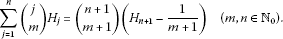(1.14)

Alzer et al. [, Eq. (3.62)] proved, by using the principle of mathematical induction, that

$\sum _{j=1}^{n}\frac{{H}_{j}}{j}=\frac{1}{2}\left[{\left({H}_{n}\right)}^{2}+{H}_{n}^{\left(2\right)}\right]\phantom{\rule{1em}{0ex}}\left(n\in \mathbb{N}\right).$
(1.15)

By using (1.15) in conjunction with the following elementary identity (see ):

${H}_{j+1}={H}_{j}+\frac{1}{j+1},$
(1.16)

we obtain

$\sum _{j=1}^{n}\frac{{H}_{j}}{j+1}=\frac{1}{2}\left[{\left({H}_{n+1}\right)}^{2}-{H}_{n+1}^{\left(2\right)}\right]\phantom{\rule{1em}{0ex}}\left(n\in \mathbb{N}\right).$
(1.17)

Chu and De Donno  made use of the classical hypergeometric summation theorems to derive several striking identities for harmonic numbers other than those discovered recently by Paule and Schneider , one of which is recalled below [, Thereoem 1].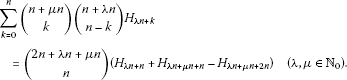(1.18)

One interesting special case of (1.18) is when we set $\mu =0$. We thus find that

$\sum _{k=0}^{n}\left(\genfrac{}{}{0}{}{n}{k}\right)\left(\genfrac{}{}{0}{}{n+\lambda n}{n-k}\right){H}_{\lambda n+k}=\left(\genfrac{}{}{0}{}{2n+\lambda n}{n}\right)\left(2{H}_{\lambda n+n}-{H}_{\lambda n+2n}\right),$
(1.19)

which can be further specialized, with $\lambda =0$, to the following form:

$\sum _{k=0}^{n}{\left(\genfrac{}{}{0}{}{n}{k}\right)}^{2}{H}_{k}=\left(\genfrac{}{}{0}{}{2n}{n}\right)\left(2{H}_{n}-{H}_{2n}\right).$
(1.20)

Dattolli and Srivastava  proposed several generating functions involving harmonic numbers by making use of an interesting approach based on the umbral calculus. Subsequently, Cvijović  showed the truth of the conjectured relations in  by using simple analytical arguments.

For a concise and beautiful description of these numbers, we refer also to WolframMathWorld’s website .

As we have seen in the above brief eclectic review, harmonic and generalized harmonic numbers are involved in a variety of useful identities. Of course, certain interesting properties of harmonic and generalized harmonic numbers have been studied (see, e.g., ). Here we aim at presenting further interesting identities about certain interesting finite series associated with binomial coefficients, harmonic numbers and generalized harmonic numbers.

## 2 Finite-series involving binomial coefficients, harmonic numbers and generalized harmonic numbers

As the illustrative identities in Section 1, we consider certain interesting identities about finite-series involving binomial coefficients, harmonic numbers and generalized harmonic numbers. We begin by recalling a known formula (cf. [, p.362, Entry (55.4.8)]; see also [, Eq. (2.6)]):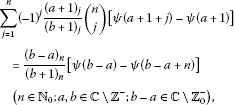(2.1)

where ${\left(\alpha \right)}_{n}$ denotes the Pochhammer symbol defined (for $\alpha \in \mathbb{C}$) by

${\left(\alpha \right)}_{n}:=\left\{\begin{array}{cc}1\hfill & \left(n=0\right),\hfill \\ \alpha \left(\alpha +1\right)\cdots \left(\alpha +n-1\right)\hfill & \left(n\in \mathbb{N}\right).\hfill \end{array}$
(2.2)

Differentiating each side of (2.1) with respect to the variables a and b, respectively, using (1.8) and considering the following easily derivable identities:

$\frac{d}{d\alpha }{\left(\alpha \right)}_{n}={\left(\alpha \right)}_{n}{H}_{n}^{\left(1\right)}\left(\alpha -1\right)\phantom{\rule{1em}{0ex}}\left(n\in {\mathbb{N}}_{0};\alpha \in \mathbb{C}\setminus {\mathbb{Z}}_{0}^{-}\right)$
(2.3)

and

$\frac{d}{d\alpha }\frac{1}{{\left(\alpha \right)}_{n}}=-\frac{{H}_{n}^{\left(1\right)}\left(\alpha -1\right)}{{\left(\alpha \right)}_{n}}\phantom{\rule{1em}{0ex}}\left(n\in {\mathbb{N}}_{0};\alpha \in \mathbb{C}\setminus {\mathbb{Z}}_{0}^{-}\right),$
(2.4)

we obtain the following formulas in Theorem 1.

Theorem 1 Each of the following identities holds true:(2.5)

and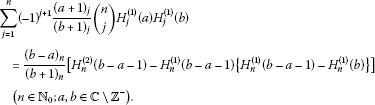(2.6)

Setting $a=b-1=0$ in (2.1), (2.5) and (2.6) and using (1.3) and (1.8), we get certain interesting finite-sum identities involving binomial coefficients and harmonic numbers, respectively, asserted by Corollary 1.

Corollary 1 Each of the following identities holds true: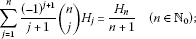(2.7)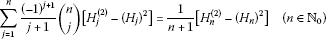(2.8)

and

$\sum _{j=1}^{n}\frac{{\left(-1\right)}^{j+1}}{j+1}\left(\genfrac{}{}{0}{}{n}{j}\right){H}_{j}\left({H}_{j+1}-1\right)=\frac{1}{n+1}\left[{H}_{n}^{\left(2\right)}-\frac{n}{n+1}{H}_{n}\right]\phantom{\rule{1em}{0ex}}\left(n\in {\mathbb{N}}_{0}\right).$
(2.9)

Remark 1 In the course of presenting a closed-form evaluation of some useful series involving the generalized zeta function $\zeta \left(s,a\right)$, Choi et al.  made use of the identity (2.7) without its proof. Choi and Srivastava  proved Eq. (2.7) as a special case of (2.1) here and presented another illustrative proof.

We will try to express a class of the following finite sums involving harmonic numbers and binomial coefficients as given above:

$\sum _{j=1}^{n}\frac{{\left(-1\right)}^{j+1}}{{\left(j+1\right)}^{k}}\left(\genfrac{}{}{0}{}{n}{j}\right){H}_{j}\phantom{\rule{1em}{0ex}}\left(n\in {\mathbb{N}}_{0};k\in \mathbb{N}\right).$
(2.10)

Here we give the answers for $k=2$ and $k=3$ in (2.10) asserted by the following lemma.

Lemma 1 Each of the following identities holds true:

$\sum _{j=1}^{n}\frac{{\left(-1\right)}^{j+1}}{{\left(j+1\right)}^{2}}\left(\genfrac{}{}{0}{}{n}{j}\right){H}_{j}=\frac{1}{2\left(n+1\right)}\left[{\left({H}_{n+1}\right)}^{2}-{H}_{n+1}^{\left(2\right)}\right]\phantom{\rule{1em}{0ex}}\left(n\in {\mathbb{N}}_{0}\right)$
(2.11)

and

$\sum _{j=1}^{n}\frac{{\left(-1\right)}^{j+1}}{{\left(j+1\right)}^{3}}\left(\genfrac{}{}{0}{}{n}{j}\right){H}_{j}=\frac{1}{2\left(n+1\right)}\sum _{j=1}^{n}\frac{1}{j+1}\left[{\left({H}_{j+1}\right)}^{2}-{H}_{j+1}^{\left(2\right)}\right]\phantom{\rule{1em}{0ex}}\left(n\in {\mathbb{N}}_{0}\right).$
(2.12)

Proof We will prove only (2.11) by using the same method as in [, pp.2224-2225]. A similar argument will establish (2.12). We first recall two basic relations for binomial coefficients:

$\left(\genfrac{}{}{0}{}{n+1}{j}\right)=\left(\genfrac{}{}{0}{}{n}{j}\right)+\left(\genfrac{}{}{0}{}{n}{j-1}\right)\phantom{\rule{1em}{0ex}}\text{and}\phantom{\rule{1em}{0ex}}\left(\genfrac{}{}{0}{}{n}{j-1}\right)=\frac{j}{n+1}\left(\genfrac{}{}{0}{}{n+1}{j}\right).$
(2.13)

We let the left-hand side of (2.11) be

${f}_{n}:=\sum _{j=1}^{n}\frac{{\left(-1\right)}^{j+1}}{{\left(j+1\right)}^{2}}\left(\genfrac{}{}{0}{}{n}{j}\right){H}_{j}$
(2.14)

so that, using the first one of (2.13),

$\begin{array}{rcl}{f}_{n+1}& =& \frac{{\left(-1\right)}^{n}}{{\left(n+2\right)}^{2}}{H}_{n+1}\\ +\sum _{j=1}^{n}\frac{{\left(-1\right)}^{j+1}}{{\left(j+1\right)}^{2}}\left[\left(\genfrac{}{}{0}{}{n}{j}\right)+\left(\genfrac{}{}{0}{}{n}{j-1}\right)\right]{H}_{j}\\ =& \frac{{\left(-1\right)}^{n}}{{\left(n+2\right)}^{2}}{H}_{n+1}+{f}_{n}+\sum _{j=1}^{n}\frac{{\left(-1\right)}^{j+1}}{{\left(j+1\right)}^{2}}\left(\genfrac{}{}{0}{}{n}{j-1}\right){H}_{j}.\end{array}$
(2.15)

We now see that, using the second one of (2.13),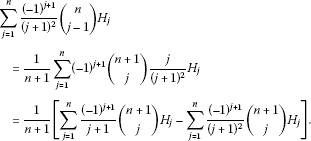(2.16)

By using the identity in (2.7), we find that

$\sum _{j=1}^{n}\frac{{\left(-1\right)}^{j+1}}{j+1}\left(\genfrac{}{}{0}{}{n+1}{j}\right){H}_{j}=\frac{1-{\left(-1\right)}^{n}}{n+2}{H}_{n+1}\phantom{\rule{1em}{0ex}}\left(n\in {\mathbb{N}}_{0}\right).$
(2.17)

We also have

$\sum _{j=1}^{n}\frac{{\left(-1\right)}^{j+1}}{{\left(j+1\right)}^{2}}\left(\genfrac{}{}{0}{}{n+1}{j}\right){H}_{j}={f}_{n+1}-\frac{{\left(-1\right)}^{n}}{{\left(n+2\right)}^{2}}{H}_{n+1}\phantom{\rule{1em}{0ex}}\left(n\in {\mathbb{N}}_{0}\right).$
(2.18)

Thus, substituting from (2.17) and (2.18) into (2.16), we obtain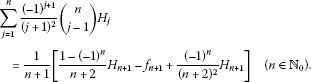(2.19)

Finally, it follows from (2.15) and (2.19) that

$\left(n+2\right){f}_{n+1}-\left(n+1\right){f}_{n}=\frac{{H}_{n+1}}{n+2}.$

Let ${a}_{n}:=\left(n+1\right){f}_{n}$ so that we have

${a}_{n+1}-{a}_{n}=\frac{{H}_{n+1}}{n+2}\phantom{\rule{1em}{0ex}}\text{and}\phantom{\rule{1em}{0ex}}{a}_{1}=\frac{1}{2}{H}_{1}=\frac{1}{2}.$
(2.20)

By telescoping this last sum (2.20), we obtain

${a}_{n}=\left(n+1\right){f}_{n}=\sum _{j=1}^{n}\frac{{H}_{j}}{j+1}.$
(2.21)

Applying (1.17) to (2.21), we get the desired identity (2.11). □

Applying (2.7) and (2.11) to (2.9) and considering (2.8), we obtain two interesting identities asserted by the following theorem.

Theorem 2 Each of the following identities holds true:

$\sum _{j=1}^{n}\frac{{\left(-1\right)}^{j+1}}{j+1}\left(\genfrac{}{}{0}{}{n}{j}\right){\left({H}_{j}\right)}^{2}=\frac{1}{2\left(n+1\right)}\left[3{H}_{n}^{\left(2\right)}-{\left({H}_{n}\right)}^{2}\right]\phantom{\rule{1em}{0ex}}\left(n\in {\mathbb{N}}_{0}\right)$
(2.22)

and

$\sum _{j=1}^{n}\frac{{\left(-1\right)}^{j+1}}{j+1}\left(\genfrac{}{}{0}{}{n}{j}\right){H}_{j}^{\left(2\right)}=\frac{1}{2\left(n+1\right)}\left[5{H}_{n}^{\left(2\right)}-3{\left({H}_{n}\right)}^{2}\right]\phantom{\rule{1em}{0ex}}\left(n\in {\mathbb{N}}_{0}\right).$
(2.23)

Differentiating (2.5) and (2.6) with respect to a and observing the following identity:

$\frac{d}{d\alpha }{H}_{j}^{\left(\ell \right)}\left(\alpha \right)=-\ell {H}_{j}^{\left(\ell \right)}\left(\alpha \right)\phantom{\rule{1em}{0ex}}\left(\ell \in \mathbb{N}\right),$
(2.24)

we obtain further interesting identities involving binomial coefficients and generalized harmonic functions asserted by the following theorem.

Theorem 3 Each of the following identities holds true: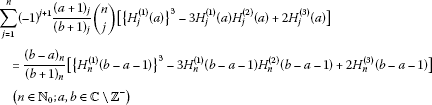(2.25)

and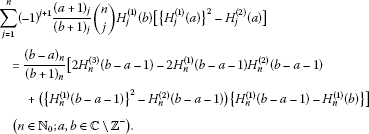(2.26)

Setting $a=b-1=0$ in (2.25) and (2.26), we find certain interesting identities and using (2.8), respectively, assert the following corollary.

Corollary 2 Each of the following identities holds true:(2.27)

and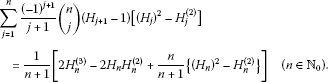(2.28)

Remark 2 As in getting the results in Theorem 3, it is seen that a variety of interesting identities involving the generalized harmonic numbers can be obtained by applying the differential operator to the parameters of known formulas.

## 3 Inverse relations and a question

By using the known orthogonal relation

$\sum _{k=j}^{n}{\left(-1\right)}^{k+j}\left(\genfrac{}{}{0}{}{n}{k}\right)\left(\genfrac{}{}{0}{}{k}{j}\right)={\delta }_{nj}\phantom{\rule{1em}{0ex}}\left(n\ge j;n,j\in {\mathbb{N}}_{0}\right),$
(3.1)

with ${\delta }_{nj}$ the Kronecker delta (${\delta }_{nn}=1$, ${\delta }_{nj}=0$ if $n\ne j$) and a manipulation of double series

$\sum _{k=0}^{n}\sum _{j=0}^{k}{A}_{k,j}=\sum _{j=0}^{n}\sum _{k=j}^{n}{A}_{k,j},$
(3.2)

it is easy to find the following simplest inverse relation (see [, Chapter 2]):

${a}_{n}=\sum _{k=0}^{n}{\left(-1\right)}^{k}\left(\genfrac{}{}{0}{}{n}{k}\right){b}_{k}\phantom{\rule{1em}{0ex}}⇔\phantom{\rule{1em}{0ex}}{b}_{n}=\sum _{k=0}^{n}{\left(-1\right)}^{k}\left(\genfrac{}{}{0}{}{n}{k}\right){a}_{k}.$
(3.3)

Applying this inverse relation to the identities in Section 2, we obtain many formulas involving binomial coefficients, harmonic numbers and generalized harmonic numbers asserted by the following corollary.

Corollary 3 Each of the following identities holds true: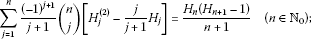(3.4)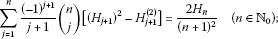(3.5)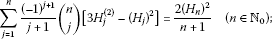(3.6)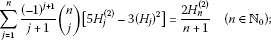(3.7)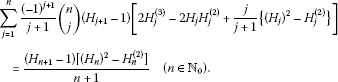(3.8)

It is observed that Eqs. (2.7), (2.8) and (2.27) are of the following form:

$\sum _{j=1}^{n}{\left(-1\right)}^{j+1}\left(\genfrac{}{}{0}{}{n}{j}\right){a}_{j}={a}_{n}\phantom{\rule{1em}{0ex}}\left(n\in \mathbb{N}\right)\phantom{\rule{1em}{0ex}}\text{and}\phantom{\rule{1em}{0ex}}{a}_{0}=0.$
(3.9)

By using the first one of (2.13), we find an identity in the following lemma.

Lemma 2 If Eq. (3.9) holds true, then we obtain the following identity:

$\sum _{j=1}^{n}{\left(-1\right)}^{j+1}j\left(\genfrac{}{}{0}{}{n}{j}\right){a}_{j}=n\left({a}_{n}-{a}_{n-1}\right)\phantom{\rule{1em}{0ex}}\left(n\in \mathbb{N}\right).$
(3.10)

Applying Eq. (3.10) to Eqs. (2.7), (2.8) and (2.27), we get some interesting identities asserted by the following corollary.

Corollary 4 Each of the following identities holds true: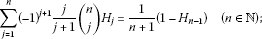(3.11)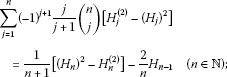(3.12)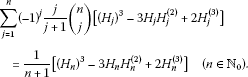(3.13)

Question We conclude this paper by posing a natural question: Under what conditions does Eq. (3.9) hold true?

## References

1. Adamchik VS, Srivastava HM: Some series of the zeta and related functions. Analysis 1998, 18: 131–144.

2. Choi J, Srivastava HM: Some summation formulas involving harmonic numbers and generalized harmonic numbers. Math. Computer Modelling 2011, 54: 2220–2234. 10.1016/j.mcm.2011.05.032

3. Graham RL, Knuth DE, Patashnik O: Concrete Mathematics. Addison-Wesley, Reading; 1989.

4. Srivastava HM, Choi J: Series Associated with the Zeta and Related Functions. Kluwer Academic, Dordrecht; 2001.

5. Srivastava HM, Choi J: Zeta and q-Zeta Functions and Associated Series and Integrals. Elsevier, Amsterdam; 2012.

6. Choi J: Certain summation formulas involving harmonic numbers and generalized harmonic numbers. Appl. Math. Comput. 2011, 218: 734–740. doi:10.1016/j.amc.2011.01.062 10.1016/j.amc.2011.01.062

7. Rassias TM, Srivastava HM: Some classes of infinite series associated with the Riemann zeta and polygamma functions and generalized harmonic numbers. Appl. Math. Comput. 2002, 131: 593–605. 10.1016/S0096-3003(01)00172-2

8. Sofo A, Srivastava HM: Identities for the harmonic numbers and binomial coefficients. Ramanujan J. 2011, 25: 93–113. 10.1007/s11139-010-9228-3

9. Coffey MW: On some series representations of the Hurwitz zeta function. J. Comput. Appl. Math. 2008, 216: 297–305. 10.1016/j.cam.2007.05.009

10. Spivey MZ: Combinatorial sums and finite differences. Discrete Math. 2007, 307: 3130–3146. 10.1016/j.disc.2007.03.052

11. Paule P, Schneider C: Computer proofs of a new family of harmonic number identities. Adv. Appl. Math. 2003, 31: 359–378. 10.1016/S0196-8858(03)00016-2

12. Schneider, C: Solving parameterized linear difference equations in ∏∑-fields. Technical Report 02–03, RISC-Linz, J. Kepler University, July 2002. Available at: http://www.risc.uni-linz.ac.at/research/combinat/risc/publications

13. Greene DH, Knuth DE: Mathematics for the Analysis of Algorithms. 3rd edition. Birkhäuser, Basel; 1990.

14. Alzer H, Karayannakis D, Srivastava HM: Series representations for some mathematical constants. J. Math. Anal. Appl. 2006, 320: 145–162. 10.1016/j.jmaa.2005.06.059

15. Chu W, De Donno L: Hypergeometric series and harmonic number identities. Adv. Appl. Math. 2005, 34: 123–137. 10.1016/j.aam.2004.05.003

16. Dattoli G, Srivastava HM: A note on harmonic numbers, umbral calculus and generating functions. Appl. Math. Lett. 2008, 21: 686–693. 10.1016/j.aml.2007.07.021

17. Cvijović D: The Dattoli-Srivastava conjectures concerning generating functions involving the harmonic numbers. Appl. Math. Comput. 2010, 215: 4040–4043. 10.1016/j.amc.2009.12.011

18. WolframMathWorld. http://mathworld.wolfram.com/HarmonicNumber.html

19. Conway JH, Guy RK: The Book of Numbers. Springer, New York; 1996.

20. Hansen ER: A Table of Series and Products. Prentice-Hall, New Jersey; 1975.

21. Choi J, Cho YJ, Srivastava HM: Series involving the zeta function and multiple gamma functions. Appl. Math. Comput. 2004, 159: 509–537. 10.1016/j.amc.2003.08.134

22. Riordan J: Combinatorial Identities. Wiley, New York; 1968.

## Acknowledgements

Dedicated to Professor Hari M. Srivastava.

This work was supported by the Basic Science Research Program through the National Research Foundation of the Republic of Korea funded by the Ministry of Education, Science and Technology (2012-0002957).

## Author information

Authors

### Corresponding author

Correspondence to Junesang Choi.

### Competing interests

The author declares that he has no competing interests.

## Rights and permissions

Reprints and Permissions

Choi, J. Finite summation formulas involving binomial coefficients, harmonic numbers and generalized harmonic numbers. J Inequal Appl 2013, 49 (2013). https://doi.org/10.1186/1029-242X-2013-49

• Accepted:

• Published:

• DOI: https://doi.org/10.1186/1029-242X-2013-49

### Keywords

• harmonic numbers
• generalized harmonic numbers
• Riemann zeta function
• Hurwitz zeta function
• Stirling numbers of the first kind
• generalized hypergeometric function ${}_{p}F_{q}$
• summation formulas for ${}_{p}F_{q}$
• psi-function
• polygamma functions